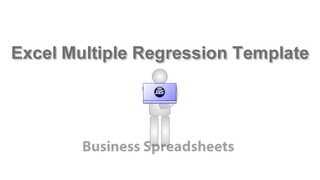## Regression Forecasting

Prediction regression equation

Write a Review!

#### What is Regression Forecasting?

Prediction regression provides the automatic identification of predictor variables through multiple regression analysis and advanced statistical tests. Regression results are presented in a simple and easy to understand format to quantify the relative influence of each input variable. The work flow facilitates and iterative process to test, maintain and discard variables until a prediction regression equation can be established with maximum confidence. Supplementary statistical analysis to reveal underlying data relationships include autocorrelation under the Dubin-Watson statistic and multicollinearity between individual independent variables. Standard tests include F statistic confidence intervals, adjusted R-squared, standard errors, t-test statistics and p values. The resulting prediction regression equation can subsequently applied to integrated forecasting methods or custom data for the independent variables to produce predictions and forecasts of desired period length. Built-in forecasting options for predictive analysis include linear, polynomial and exponential methodologies. Prediction regression runs in Excel for both Mac and Windows platforms.

### Regression Forecasting Overview

Starting from
US\$14,00

Free Trial

USD 14.00

#### Regression Forecasting Features

Devices
S M L
Markets
United States, Canada, United Kingdom, Australia, China and 3 others
Supported Languages
English

### MediaStarting from
US\$14,00
Free Trial

USD 14.00

### Regression Forecasting Features

API
Activity Dashboard
Charting
Collaboration Tools
Customizable Reporting
Dashboard Creation
Data Filtering
Data Import/Export
Data Visualization
Monitoring
Multiple Data Sources
Predictive Analytics
Real Time Analytics
Real Time Data
Reporting & Statistics
Third Party Integration
Trend Analysis
Visual Analytics

### Additional information for Regression Forecasting

#### Key features of Regression Forecasting

• Accommodates any type of business or scientific data
• Input data verification and cleaning
• Options to employ polynomial, exponential or linear trends
• Prediction is based on statistical level of confidence.
• Regression prediction equation is used for forecasting data
• Regression statistics for each independent variable
• Results are displayed in user friendly explanations
• Simple and intuitive workflow
• Standard errors t-statistics and p-values
• Tests significance, autocorrelation and multicollinearity

#### Benefits

The regression prediction application is a fast and easy to use solution for forecasting and identifying causal relationships.

### Regression Forecasting FAQs

Below are some frequently asked questions for Regression Forecasting.

#### Q. What type of pricing plans does Regression Forecasting offer?

Regression Forecasting offers the following pricing plans:

Starting from: US\$14,00

Free Trial: Available

USD 14.00

#### Q. What are the main features of Regression Forecasting?

Regression Forecasting offers the following features:

• Accommodates any type of business or scientific data
• Input data verification and cleaning
• Options to employ polynomial, exponential or linear trends
• Prediction is based on statistical level of confidence.
• Regression prediction equation is used for forecasting data
• Regression statistics for each independent variable
• Results are displayed in user friendly explanations
• Simple and intuitive workflow
• Standard errors t-statistics and p-values
• Tests significance, autocorrelation and multicollinearity

#### Q. Who are the typical users of Regression Forecasting?

Regression Forecasting has the following typical customers:

#### Q. What languages does Regression Forecasting support?

Regression Forecasting supports the following languages:

English

#### Q. What type of pricing plans does Regression Forecasting offer?

Regression Forecasting has the following pricing plans:

#### Q. Does Regression Forecasting support mobile devices?

We do not have any information about what devices Regression Forecasting supports

#### Q. What other apps does Regression Forecasting integrate with?

We do not have any information about what integrations Regression Forecasting has

#### Q. What level of support does Regression Forecasting offer?

Regression Forecasting offers the following support options:

FAQs, Forum, Online Support, Video Tutorials